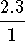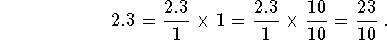#Rule 28: Converting Decimals to FractionsFor this review, we will focus on terminating fractions. Divide the fraction by 1, and then multiply the result by 1 in a form that will remove the decimal.Example: Convert 2.3 to a fraction.

Answer. 2.3 can be writtenwhich can be multiplied by 1 and divided by 1 without changing its value. We have### If you would like to review another example, click on Example.Work the following problems and click on Answer if you want to check the answer and/or review the solution.

Problem 1: Convert 0.00235 to a simple fraction.

Problem 2: Convert 4.28 to a simple fraction and to a compound number.

Problem 3: Convert 10.0000255 to a simple fraction and to a compound number.[Simple Fractions] [Complex Fractions] [Compound Fractions]
[Decimals] [Percentage] [Rules]

[Algebra] [Trigonometry] [Complex Variables]S.O.S MATHematics home page

Do you need more help? Please post your question on our S.O.S. Mathematics CyberBoard.Author: Nancy Marcus